6248

Mathematics Coordinate Geometry Level: Misc Level

Looking for the midpoint between the line segment of the points these 2 problems:

1) P1=(-4,-3) andP2=(2,2) (2) P1=(2,-3) and P2=(1,-2)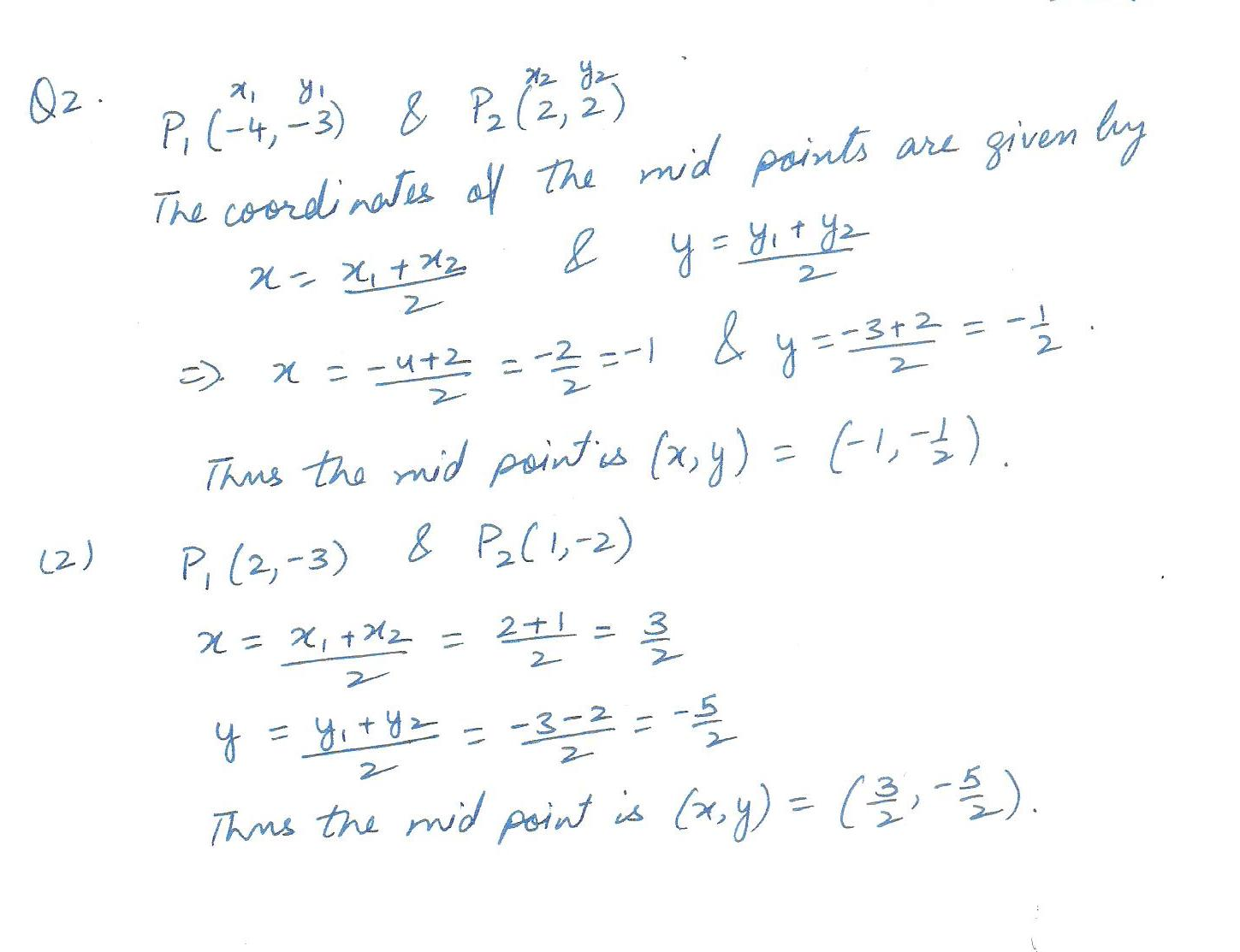6247

Mathematics Coordinate Geometry Level: Misc Level

Looking for the distance between the points on the first two problems:1) P1=(-4,-1) and P2= (-2,-5) P1 =( 2,-3) p2=(4,2)5413

Mathematics Coordinate Geometry Level: Misc Level

Draw the graphs, in the coordinate plane, of the conditions stated: { (x,y) : y2 >=2y}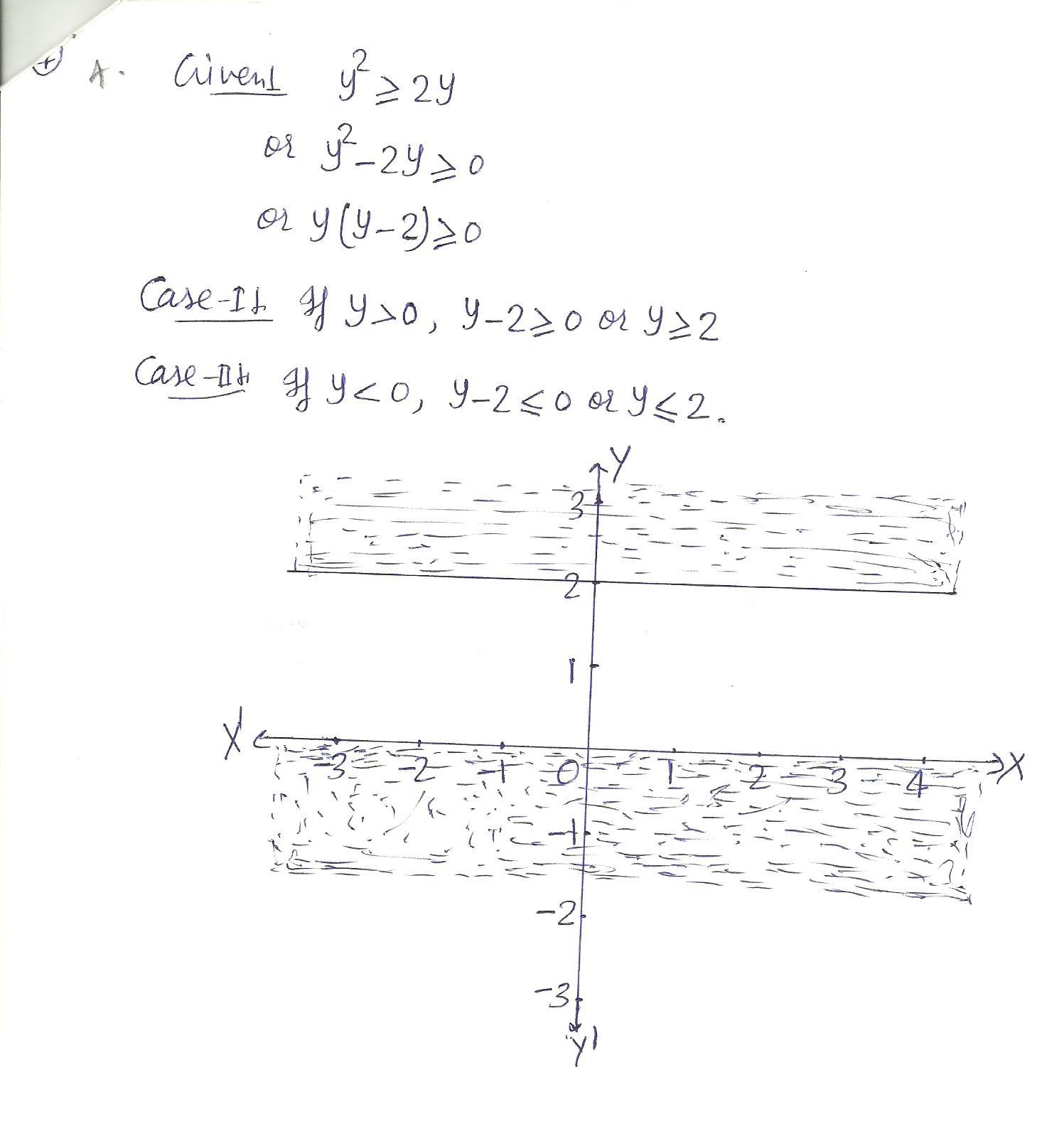5412

Mathematics Coordinate Geometry Level: Misc Level

Draw the graphs, in the coordinate plane, of the conditions stated: { (x,y ): y2-3y < 0 }

(y2 is y to the power of 2)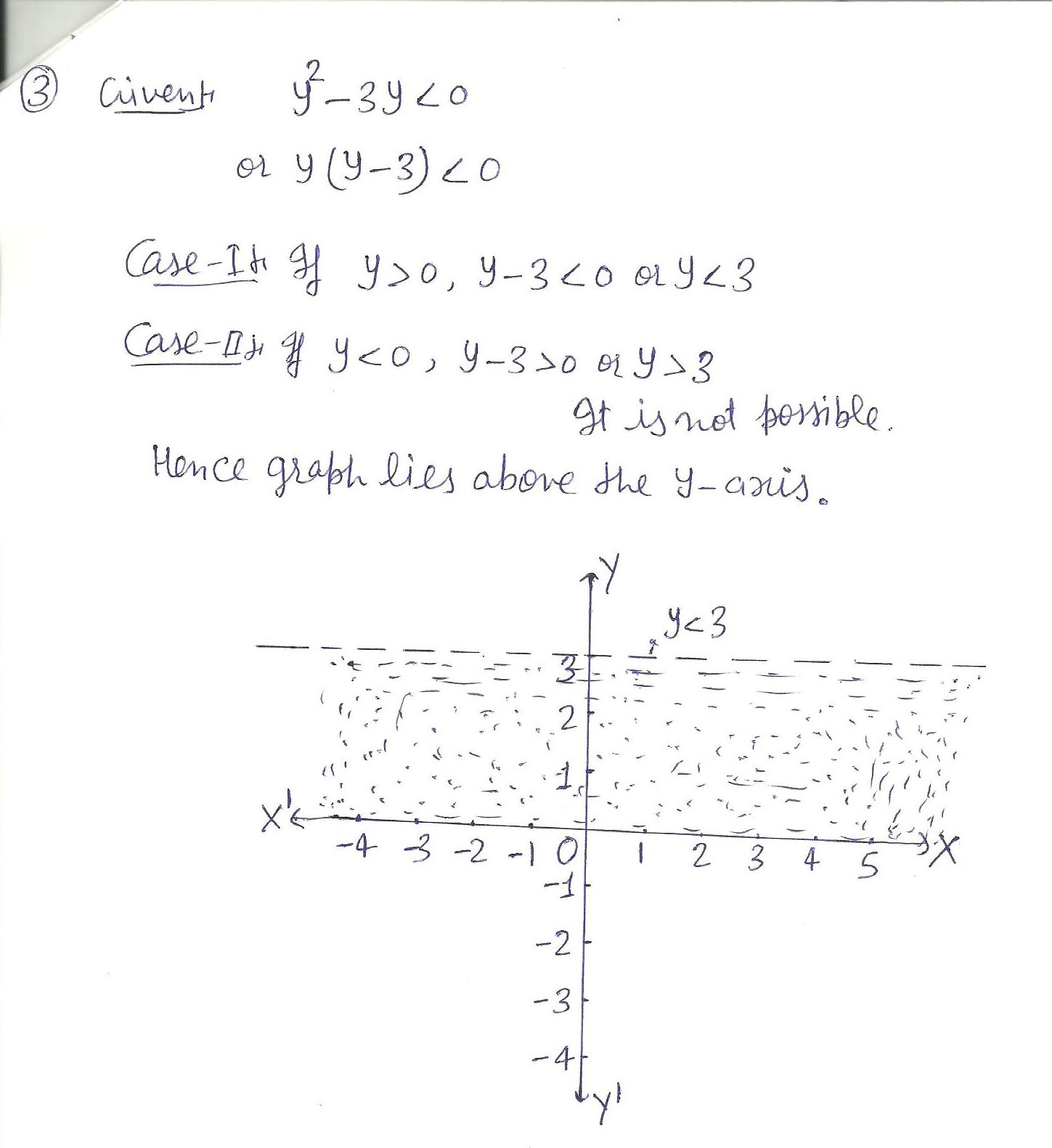5411

Mathematics Coordinate Geometry Level: Misc Level

Five of the vertices of a rectangular solid are points (-1,1,-5),(-1,1,2)(-1,3,2), (-13,-5), and (1,-1,-5). State the coordinates of the other three vertices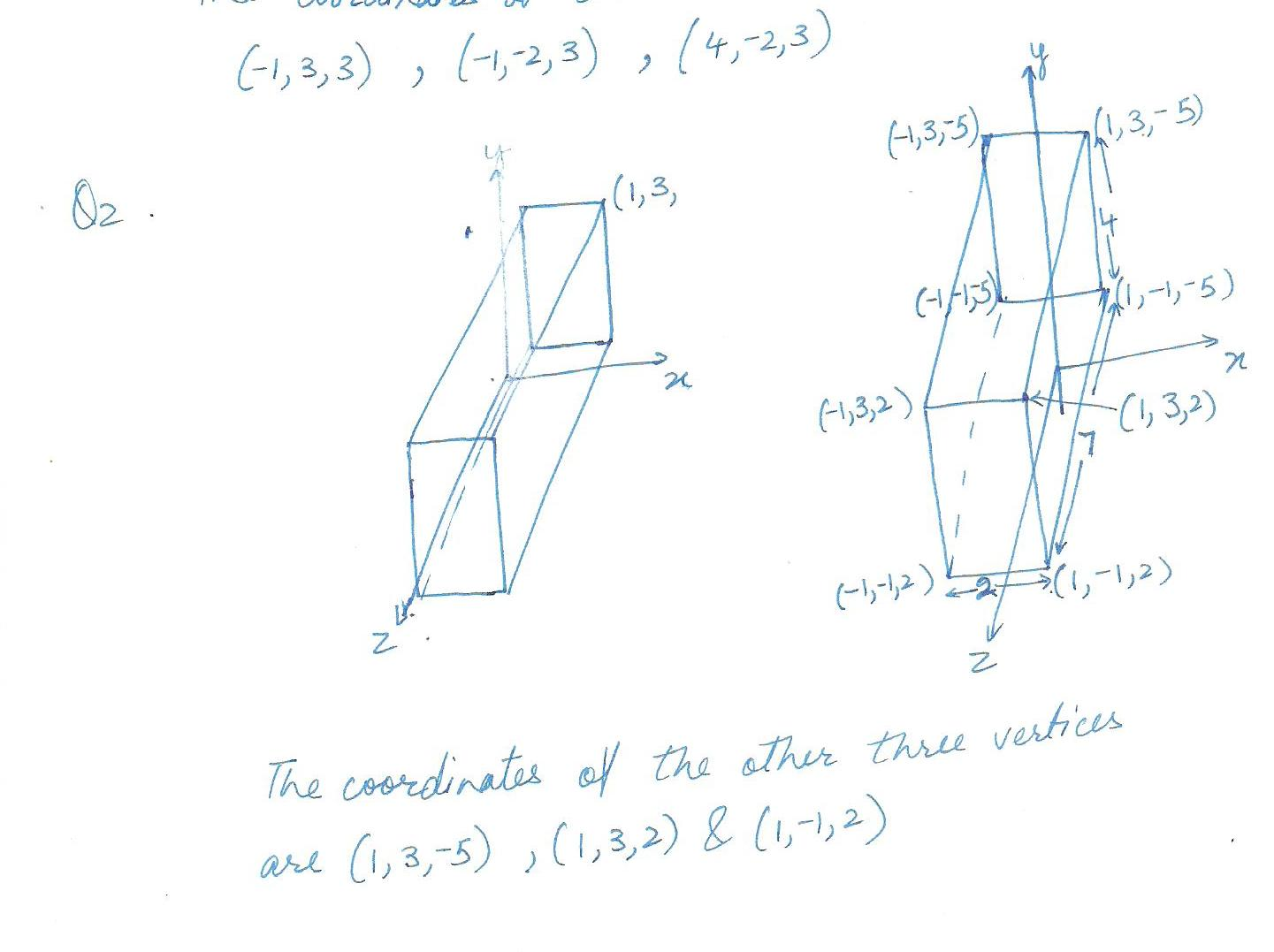5410

Mathematics Coordinate Geometry Level: Misc Level

five on the eight vertices of a cube are points ( -1,3,-2), (4,3,-2), (4,-2,-2),(-1,-2,-2), and (4,3,3). State the coordinates of the other three vertices.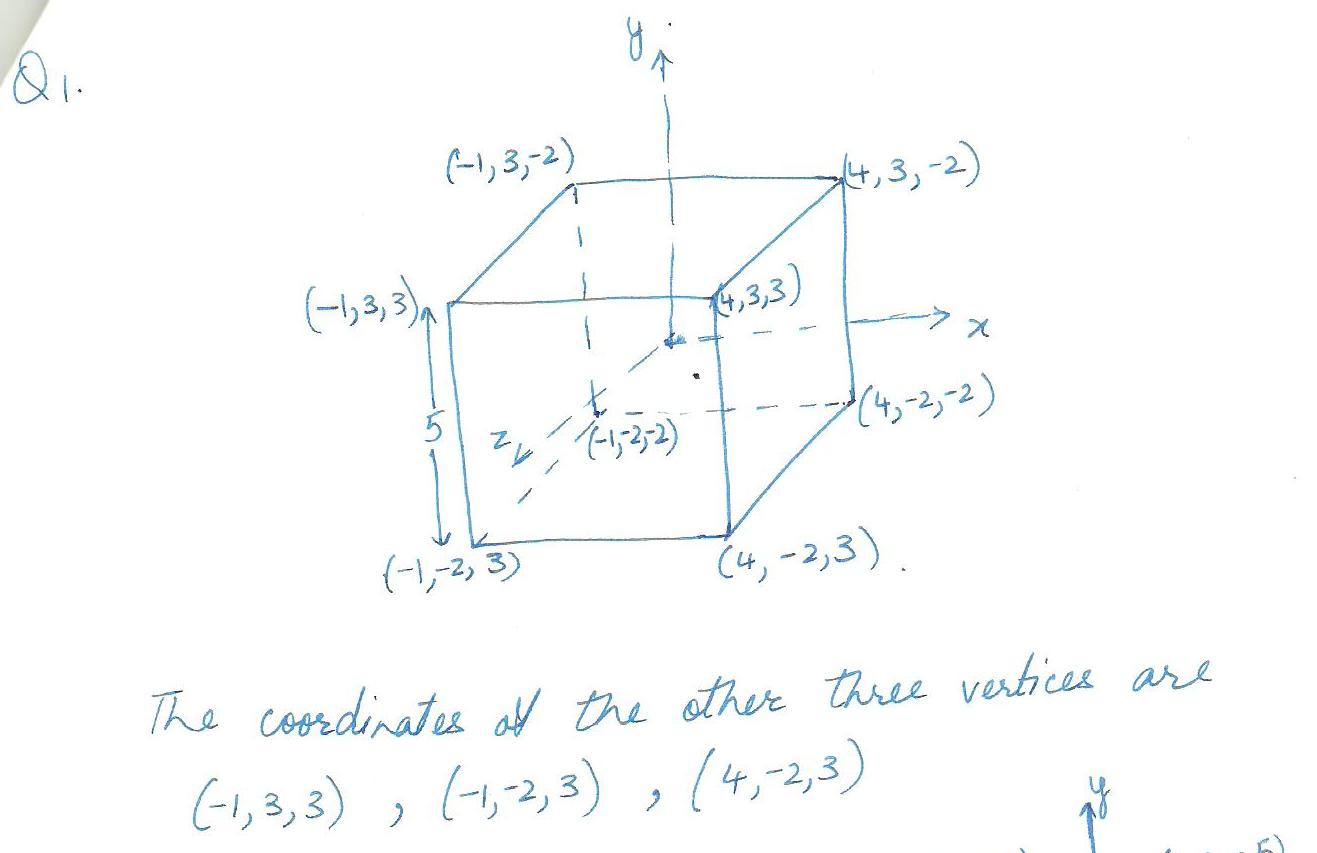4939

Mathematics Coordinate Geometry Level: Misc Level

What is the angle between the line L: x =1 +2t, y=2-t, z=-2t and the plane whose equation is 3x +2y+z =5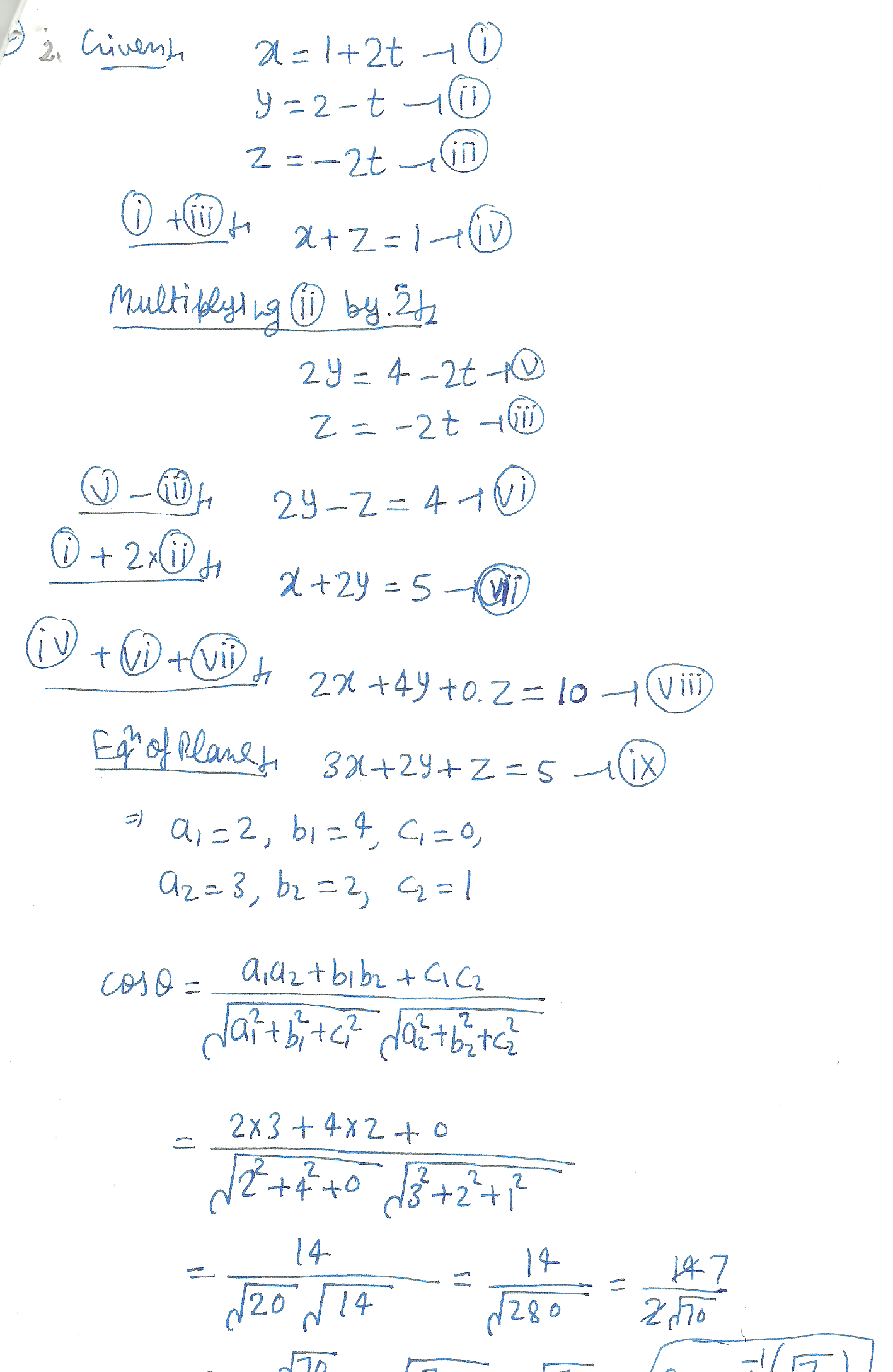3192

Mathematics Coordinate Geometry Level: High School

a ) Find the slope of any line parallel to the line through points (18, 14) and (10, 10).
b ) Find the slope of any line perpendicular to the line through points (11, 3) and (9, 7).3191

Mathematics Coordinate Geometry Level: High School

Slope and the y intercept of a line.

Find the slope and the y-intercept.
y = â€“7x + 3
Find the slope and y-intercept.
10x + 6y = â€“543190

Mathematics Coordinate Geometry Level: High School

Drawing the graph of a line in the form y=mx + c where m is the slope of the graph and c is the y-axis intercept.

Graph y = -2x + 1.3189

Mathematics Coordinate Geometry Level: High School

Constant of variation of a line

If y varies directly with x, and y = 144 when x = 8, find the constant of variation k.3188

Mathematics Coordinate Geometry Level: High School

Slope of a line passing through two points.

Find the slope of the line passing through the points (2, 1) and (4, 7).
Find the slope of the line passing through the points (3, -5) and (3, 1).3187

Mathematics Coordinate Geometry Level: High School

Coordinate Geometry

Complete the ordered pairs for the equation 2x + y = 10.
(5, ) , ( , 10) , ( , - 2) , (0, )Displaying 1-13 of 13 results.